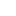v2.28IPlacement3D Interface Reference

Represents a local coordinate system in three-dimensional space. More...

Inheritance diagram for IPlacement3D:## Public Member Functions

HRESULT GetCopy ([out, retval] IPlacement3D **ppCopy)
Returns a copy of the coordinate system.

HRESULT GetTransformFrom ([out, retval] ITransform3D **ppTransform)
Returns the transform from the current coordinate system to the global (in relation to the current) coordinate system.

HRESULT GetTransformInto ([out, retval] ITransform3D **ppTransform)
Returns the transform from the global (in relation to the current) coordinate system to the current coordinate system.

HRESULT IsLeft ([out, retval] VARIANT_BOOL *pResult)
Checks whether the local coordinate system is left.

HRESULT IsNormal ([out, retval] VARIANT_BOOL *pResult)
Checks whether the local coordinate system is normal.

HRESULT IsOrthogonal ([out, retval] VARIANT_BOOL *pResult)
Checks whether the local coordinate system is orthogonal.

HRESULT Move ([in] Vector3D vector_)
Moves the coordinate system by the given vector.

HRESULT Rotate ([in] Vector3D axis_, [in] double angle_)
Rotates the coordinate system to a given angle around the given axis.

HRESULT Transform ([in] ITransform3D *pTransform)
Transformes the coordinate system according to a given transform.

## Properties

Vector3D AxisX` [get]`
Returns the X axis vector of the coordinate system.

Vector3D AxisY` [get]`
Returns the Y axis vector of the coordinate system.

Vector3D AxisZ` [get]`
Returns the Z axis vector of the coordinate system.

Point3D Origin` [get]`
Returns the origin of the coordinate system.

## Detailed Description

Represents a local coordinate system in three-dimensional space.

A local coordinate system is described by an origin point and three non-collinear axis vectors.